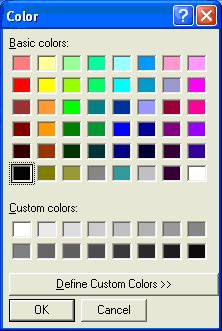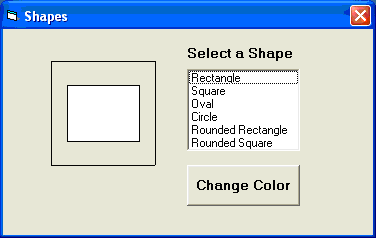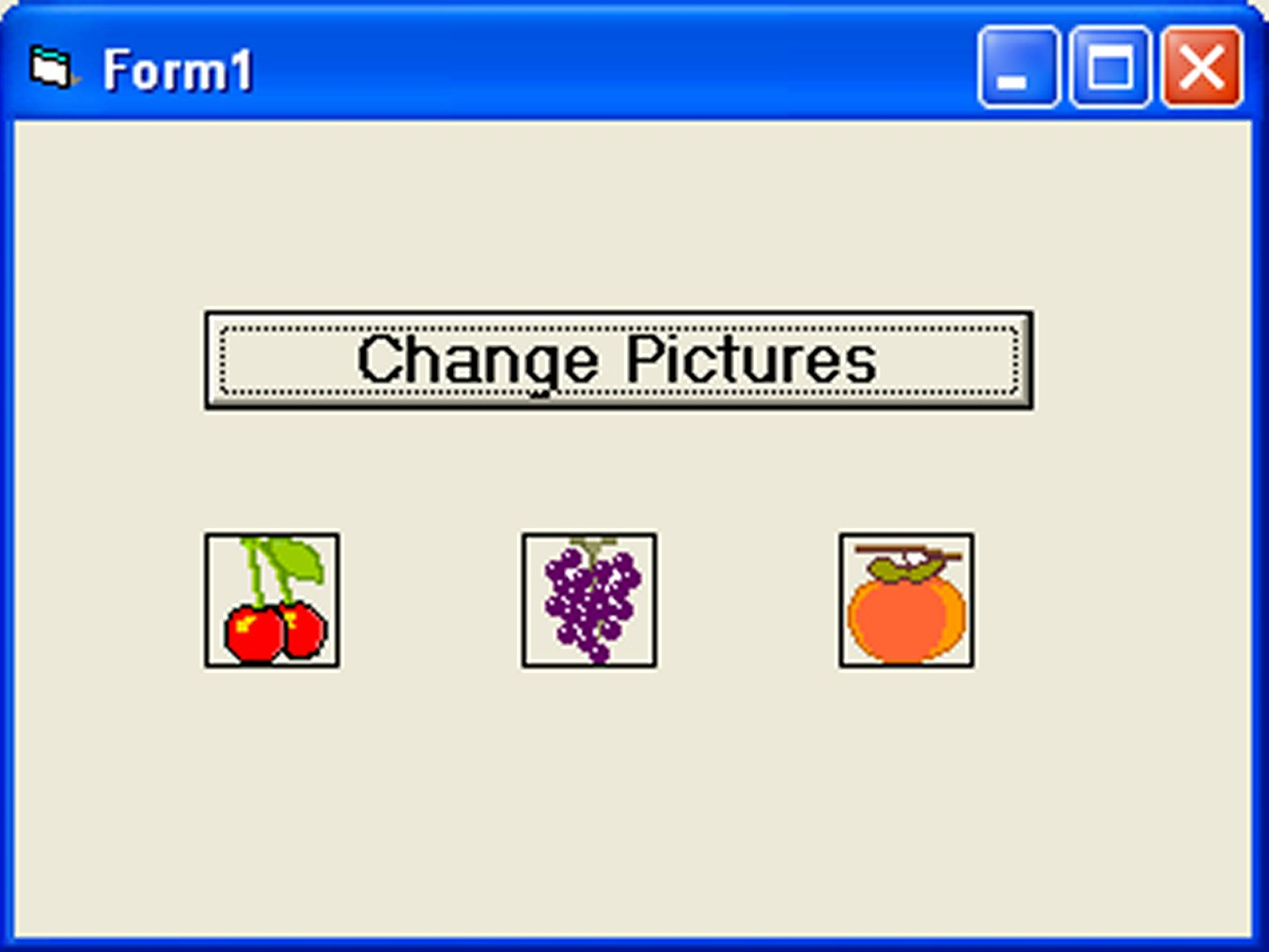# Lesson 18 : Creating Graphics

Graphics forms a very important part of visual basic programming because an attractive user interface will be appealing to the users. In the old BASIC, drawing and designing graphics are considered difficult jobs, as they have to be programmed line by line in a text-based environment. However, in Visual Basic 6, these jobs have been made easy. There are four basic controls in VB6 that you can use to draw graphics on your form: the line control, the shape control, the image box and the picture box

### 18.1 The line and Shape controls

To draw a straight line, just click on the line control and then use your mouse to draw the line on the form. After drawing the line, you can then change its color, width and style using the BorderColor, BorderWidth and BorderStyle properties.Similarly, to draw a shape, just click on the shape control and draw the shape on the form. The default shape is a rectangle, with the default shape property set at 0. You can change the shape to square, oval, circle and rounded rectangle by changing the shape property’s value to 1, 2, 3 , 4, and 5 respectively. In addition, you can change its background color using the BackColor property, its border style using the BorderStyle property, its border color using the BorderColor property as well its border width using the BorderWidth property.

#### Example 18.1

The program in this example allows the user to change the shape by selecting a particular shape from a list of options from a list box, as well as changing its color through a common dialog box.

The objects to be inserted in the form are a list box, a command button, a shape control and a common dialog box. The common dialog box can be inserted by clicking on ‘project’ on the menu and then select the Microsoft Common Dialog Control 6.0 by clicking the check box. After that, the Microsoft Common Dialog Control 6.0 will appear in the toolbox; and you can drag it into the form. The list of items can be added to the list box through the AddItem method. The procedure for the common dialog box to present the standard colors is as follows:

```
CommonDialog1.Flags = &H1&
CommonDialog1.ShowColor
Shape1.BackColor = CommonDialog1.Color

```

The last line will change the background color of the shape by clicking on a particular color on the common dialog box as shown in the Figure 18.1 below:

### The Code

```Private Sub Form_Load()
End Sub```
```Private Sub List1_Click()
Select Case List1.ListIndex
Case 0
Shape1.Shape = 0
Case 1
Shape1.Shape = 1
Case 2
Shape1.Shape = 2
Case 3
Shape1.Shape = 3
Case 4
Shape1.Shape = 4
Case 5
Shape1.Shape = 5
End Select
End Sub
```
```Private Sub Command1_Click()
CommonDialog1.Flags = &H1&
CommonDialog1.ShowColor
Shape1.BackColor = CommonDialog1.Color
End Sub
```#### Figure 18.1 The color dialog box### 18.2 The Image Box and the Picture Box

Using the line and shape controls to draw graphics will only enable you to create a simple design. In order to improve the look of the interface, you need to put in images and pictures of your own. Fortunately, there are two very powerful graphics tools you can use in Visual Basic 6 which are the image box and the picture box.

To load a picture or image into an image box or a picture box, you can click on the picture property in the properties window to launch a dialog box that will prompt you to select a certain picture file. You can also load a picture at runtime by using the LoadPictrure ( ) method. The syntax is

```Image1.Picture= LoadPicture("C:\pathname\picture file name")

For example, the following statement will load the grape.gif picture into the image box.

`Image1.Picture= LoadPicture("C:\MyFolder\VB program\Images\grape.gif")`

#### Example 18.2

In this example, each time you click on the ‘change pictures’ button as shown in Figure 18.2, you will be able to see three images loaded into the image boxes. This program uses the Rnd function to generate random integers and then uses the LoadPicture method to load different pictures into the image boxes using the If…Then…Statements based on the random numbers generated.

#### The code

```
Dim a, b, c As Integer
Private Sub Command1_Click ()
a = 3 + Int(Rnd * 3)
b= 3 + Int(Rnd * 3)
c= 3 + Int(Rnd * 3)
If a = 3 Then
End If
If a = 4 Then
End If
If a = 5 Then
End If
If b = 3 Then
End If
If b = 4 Then
End If
If b = 5 Then
End If
If c = 3 Then
End If
If c = 4 Then
End If
If c = 5 Then
End If
End Sub```### 18.3 PSet, Line and Circle Drawing Methods

Other than using the line and shape controls to draw graphics on the form, you can also use the Pset, Line and Circle methods to draw graphics on the form.

#### (a) The Pset Method

The Pset method draw a dot on the screen, it takes the syntax

Pset (x , y ), color

(x,y) is the coordinates of the point and color is its color. To specify the color, you can use the color codes or the standard VB color constant such as VbRed, VbBlue, VbGeen and etc. For example, Pset(100,200), VbRed will display a red dot at the (100,200) coordinates.

The Pset method can also be used to draw a straight line on the form. The procedure is

```For x= a to b
Pset(x,x)
Next x
```

This procedure will draw a line starting from the point (a,a) and to the point (b,b). For example, the following procedure will draw a magenta line from the point (0,0) to the point (1000,1000).

```For x= 0 to 100
Pset(x,x) , vbMagenta
Next x
```

#### (b) The Line Method

Although the Pset method can be used to draw a straight line on the form, it is a little slow. It is better to use the Line method if you want to draw a straight line faster. The format of the Line command is shown below. It draws a line from the point (x1, y1) to the point (x2, y2) and the color constant will determine the color of the line.

`Line (x1, y1)-(x2, y2), color`

For example, the following command will draw a red line from the point (0, 0) to the point (1000, 2000).

`Line (0, 0)-(1000,2000), VbRed `

The Line method can also be used to draw a rectangle. The syntax is

`Line (x1-y1)-(x2, y2), color, B`

The four corners of the rectangle are (x1-y1), (x2-y1), (x1-y2) and (x2, y2)

Another variation of the Line method is to fill the rectangle with a certain color. The syntax is

`Line (x1, y1)-(x2, y2), color, BF`

If you wish to draw the graphics in a picture box, you can use the following syntaxes

```
Picture1.Line (x1, y1)-(x2, y2), color
Picture1.Line (x1-y1)-(x2, y2), color, B
Picture1.Line (x1-y1)-(x2, y2), color, BF
```

#### (c) The Circle Method

The circle method uses the following syntax

`Circle (x1, y1),radius, color`

to draws a circle centered at (x1, y1), with a certain radius and a certain border color.

For example, the procedure

`Circle (400, 400),500, VbRed`

draws a circle centered at (400, 400) with a radius of 500 twips and a red border.